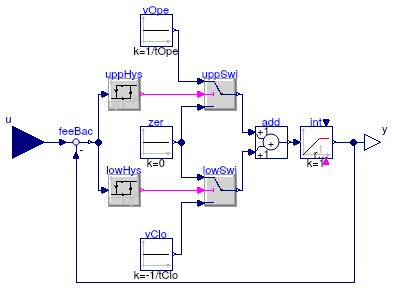## Buildings.Fluid.Actuators.Motors

Package with motor models for valves and dampers

### Information

This package contains component models for motors that may be used with the actuator models in Buildings.Fluid.Actuators.Dampers or Buildings.Fluid.Actuators.Valves.

Extends from Modelica.Icons.VariantsPackage (Icon for package containing variants).

### Package Content

Name DescriptionIdealMotor Ideal motor model with hysteresisExamples Collection of models that illustrate model use and test models

##Buildings.Fluid.Actuators.Motors.IdealMotor

Ideal motor model with hysteresis### Information

Ideal actuator motor model with hysteresis and finite actuation speed. If the current actuator position `y` is below (or above) the input signal `u` by an amount bigger than the hysteresis `delta`, then the position `y` is increased (decreased) until it reaches `u`. The output `y` is bounded between `0` and `1`.

Note: This model can introduce state events which increase the computation time. For a more efficient implementation that approximates a motor, set in the valve or damper model the parameter `use_inputFilter=true` instead of using this motor model. See also Buildings.Fluid.Actuators.UsersGuide.

Extends from Modelica.Blocks.Interfaces.SISO (Single Input Single Output continuous control block).

### Parameters

TypeNameDefaultDescription
Realdelta0.05Hysteresis
TimetOpe120Opening time [s]
TimetClotOpeClosing time [s]
Realy_start0.5Start position

### Connectors

TypeNameDescription
input RealInputuConnector of Real input signal
output RealOutputyConnector of Real output signal

### Modelica definition

model IdealMotor "Ideal motor model with hysteresis" extends Modelica.Blocks.Interfaces.SISO; parameter Real delta(min=0, max=0.5) = 0.05 "Hysteresis"; parameter Modelica.SIunits.Time tOpe(min=0) = 120 "Opening time"; parameter Modelica.SIunits.Time tClo(min=0) = tOpe "Closing time"; parameter Real y_start(min=0, max=1) = 0.5 "Start position"; Modelica.Blocks.Logical.Hysteresis uppHys(final uLow=0, uHigh=delta, final pre_y_start=false); Modelica.Blocks.Logical.Hysteresis lowHys(uLow=-delta, final uHigh=0, final pre_y_start=true) "Lower hysteresis"; Modelica.Blocks.Logical.Switch uppSwi; Modelica.Blocks.Continuous.LimIntegrator int( final y_start=y_start, final k=1, outMax=1, outMin=0, initType=Modelica.Blocks.Types.Init.InitialState, limitsAtInit=true, y(stateSelect=StateSelect.always)) "Integrator for valve opening position"; protected final Modelica.Blocks.Sources.Constant zer(final k=0) "Zero signal"; Modelica.Blocks.Sources.Constant vOpe(final k=1/tOpe) "Opening speed"; Modelica.Blocks.Sources.Constant vClo(final k=-1/tClo) "Closing speed"; Modelica.Blocks.Logical.Switch lowSwi; Modelica.Blocks.Math.Add add; Modelica.Blocks.Math.Feedback feeBac "Feedback to compute position error"; equation connect(zer.y, uppSwi.u3); connect(uppHys.y, uppSwi.u2); connect(vOpe.y, uppSwi.u1); connect(lowHys.y, lowSwi.u2); connect(vClo.y, lowSwi.u3); connect(zer.y, lowSwi.u1); connect(add.y, int.u); connect(uppSwi.y, add.u1); connect(u, feeBac.u1); connect(feeBac.y, uppHys.u); connect(feeBac.y, lowHys.u); connect(lowSwi.y, add.u2); connect(int.y, y); connect(int.y, feeBac.u2); end IdealMotor;